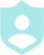# Solving Quadratics By Completing the Square 4###### Example QuestionsSolve each equation by completing the square.Solve each equation by completing the square.
##### Description :
Solve Quadratic Equations using the Complete the Square technique.##### Difficulty :TRIVIA 10 QuestionsDownloadPrintShareAdd
Questions

•Solve each equation by completing the square.Colors :
Font color
Button color
Background color
Logo:Background Image:Theme Song: